# Centripetal force

﻿
Centripetal forceRoller coaster cars are forced through a loop by the track applying a centripetal force on them. A reactive centrifugal force is applied to the track by the cars.

Centripetal force (from Latin centrum "center" and petere "to seek") is a force that makes a body follow a curved path: it is always directed orthogonal to the velocity of the body, toward the instantaneous center of curvature of the path. The mathematical description was derived in 1659 by Dutch physicist Christiaan Huygens. Isaac Newton's description was: "A centripetal force is that by which bodies are drawn or impelled, or in any way tend, towards a point as to a center."

## Formula

The magnitude of the centripetal force on an object of mass m moving at a speed v along a path with radius of curvature r is:$F = ma_c = \frac{m v^2}{r}$

where ac is the centripetal acceleration. The direction of the force is toward the center of the circle in which the object is moving, or the osculating circle, the circle that best fits the local path of the object, if the path is not circular. This force is also sometimes written in terms of the angular velocity ω of the object about the center of the circle:$F = m r \omega^2 \,$

Expressed using the period for one revolution of the circle, T, the equation becomes:$F = m \frac{4\pi^2r}{T^2}.$

## Sources of centripetal force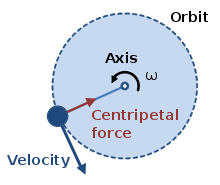A body experiencing uniform circular motion requires a centripetal force, towards the axis as shown, to maintain its circular path.

For a satellite in orbit around a planet, the centripetal force is supplied by gravity. Some sources, including Newton, refer to the entire force as a centripetal force, even for non-circular orbits, for which gravity is not aligned with the direction to the center of curvature.

The gravitational force acts on each object toward the other, which is toward the center of mass of the two objects; for circular orbits, this center of gravity is the center of the circular orbits. For non-circular orbits or trajectories, only the component of gravitational force directed orthogonal to the path (toward the center of the osculating circle) is termed centripetal; the remaining component acts to speed up or slow down the satellite in its orbit. For an object swinging around on the end of a rope in a horizontal plane, the centripetal force on the object is supplied by the tension of the rope. For a spinning object, internal tensile stress provides the centripetal forces that make the parts of the object trace out circular motions.

The rope example is an example involving a 'pull' force. The centripetal force can also be supplied as a 'push' force such as in the case where the normal reaction of a wall supplies the centripetal force for a wall of death rider.

Another example of centripetal force arises in the helix which is traced out when a charged particle moves in a uniform magnetic field in the absence of other external forces. In this case, the magnetic force is the centripetal force which acts towards the helix axis.

## Analysis of several cases

Below are three examples of increasing complexity, with derivations of the formulas governing velocity and acceleration.

### Uniform circular motion

Uniform circular motion refers to the case of constant rate of rotation. Here are two approaches to describing this case.

#### Calculus derivation

In two dimensions the position vector$\textbf{r}$ which has magnitude (length) r and directed at an angle θ above the x-axis can be expressed in Cartesian coordinates using the unit vectors$\hat{x}$ and$y-hat$:$\textbf{r} = r \cos(\theta) \hat{x} + r \sin(\theta) \hat{y}$

Assume uniform circular motion, which requires three things.

1. The object moves only on a circle.
2. The radius of the circle r does not change in time.
3. The object moves with constant angular velocity ω around the circle. Therefore θ = ωt where t is time.

Now find the velocity$\textbf{v}$ and acceleration$\textbf{a}$ of the motion by taking derivatives of position with respect to time.$\textbf{r} = r \cos(\omega t) \hat{x} + r \sin(\omega t) \hat{y}$$\dot{\textbf{r}} = \textbf{v} = - r \omega \sin(\omega t) \hat{x} + r \omega \cos(\omega t) \hat{y}$$\ddot{\textbf{r}} = \textbf{a} = - r \omega^2 \cos(\omega t) \hat{x} - r \omega^2 \sin(\omega t) \hat{y}$$\textbf{a} = - \omega^2 (r \cos(\omega t) \hat{x} + r \sin(\omega t) \hat{y})$

Notice that the term in parenthesis is the original expression of$\textbf{r}$ in Cartesian coordinates. Consequently,$\textbf{a} = - \omega^2 \textbf{r}$

The negative shows that the acceleration is pointed towards the center of the circle (opposite the radius), hence it is called "centripetal" (i.e. "center-seeking"). While objects naturally follow a straight path (due to inertia), this centripetal acceleration describes the circular motion path caused by a centripetal force.

#### Derivation using vectors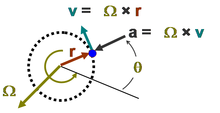Figure 3: Vector relationships for uniform circular motion; vector Ω representing the rotation is normal to the plane of the orbit with polarity determined by the right-hand rule and magnitude /dt.

Figure 3 shows the vector relationships for uniform circular motion. The rotation itself is represented by the vector Ω, which is normal to the plane of the orbit (using the right-hand rule) and has magnitude given by:$|\mathbf{\Omega}| = \frac {\mathrm{d} \theta } {\mathrm{d}t} = \omega \ ,$

with θ the angular position at time t. In this subsection, dθ/dt is assumed constant, independent of time. The distance traveled of the particle in time dt along the circular path is$\mathrm{d}\boldsymbol{\ell} = \mathbf {\Omega} \times \mathbf{r}(t) \mathrm{d}t \ ,$

which, by properties of the vector cross product, has magnitude rdθ and is in the direction tangent to the circular path.

Consequently,$\frac {\mathrm{d} \mathbf{r}}{\mathrm{d}t} = \frac {\mathbf{r}(t + \mathrm{d}t)-\mathbf{r}(t)}{\mathrm{d}t} = \frac{\mathrm{d} \boldsymbol{\ell}}{\mathrm{d}t} \ .$

In other words,$\mathbf{v}\ \stackrel{\mathrm{def}}{ = }\ \frac {\mathrm{d} \mathbf{r}}{\mathrm{d}t} = \frac {\mathrm{d}\mathbf{\boldsymbol{\ell}}}{\mathrm{d}t} = \mathbf {\Omega} \times \mathbf{r}(t)\ .$

Differentiating with respect to time,$\mathbf{a}\ \stackrel{\mathrm{def}}{ = }\ \frac {\mathrm{d} \mathbf{v}} {d\mathrm{t}} = \mathbf {\Omega} \times \frac{\mathrm{d} \mathbf{r}(t)}{\mathrm{d}t} = \mathbf{\Omega} \times \left[ \mathbf {\Omega} \times \mathbf{r}(t)\right] \ .$

Lagrange's formula states:$\mathbf{a} \times \left ( \mathbf{b} \times \mathbf{c} \right ) = \mathbf{b} \left ( \mathbf{a} \cdot \mathbf{c} \right ) - \mathbf{c} \left ( \mathbf{a} \cdot \mathbf{b} \right ) \ .$

Applying Lagrange's formula with the observation that Ω • r(t) = 0 at all times,$\mathbf{a} = - {|\mathbf{\Omega|}}^2 \mathbf{r}(t) \ .$

In words, the acceleration is pointing directly opposite to the radial displacement r at all times, and has a magnitude:$|\mathbf{a}| = |\mathbf{r}(t)| \left ( \frac {\mathrm{d} \theta}{\mathrm{d}t} \right) ^2 = R {\omega}^2\$

where vertical bars |...| denote the vector magnitude, which in the case of r(t) is simply the radius R of the path. This result agrees with the previous section, though the notation is slightly different.

When the rate of rotation is made constant in the analysis of nonuniform circular motion, that analysis agrees with this one.

A merit of the vector approach is that it is manifestly independent of any coordinate system.

#### Example: The banked turn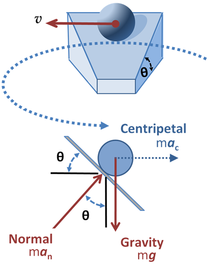Figure 4: Upper panel: Ball on a banked circular track moving with constant speed v; Lower panel: Forces on the ball.

The upper panel in Figure 4 shows a ball in circular motion on a banked curve. The curve is banked at an angle θ from the horizontal, and the surface of the road is considered to be slippery. The object is to find what angle the bank must have so the ball does not slide off the road. Intuition tells us that on a flat curve with no banking at all, the ball will simply slide off the road; while with a very steep banking, the ball will slide to the center unless it travels the curve rapidly.

Apart from any acceleration that might occur in the direction of the path, the lower panel of Figure 4 indicates the forces on the ball. There are two forces; one is the force of gravity vertically downward through the center of mass of the ball mg where m is the mass of the ball and g is the gravitational acceleration; the second is the upward normal force exerted by the road perpendicular to the road surface man. The centripetal force demanded by the curved motion also is shown in Figure 4. This centripetal force is not a third force applied to the ball, but rather must be provided by the net force on the ball resulting from vector addition of the normal force and the force of gravity. The resultant or net force on the ball found by vector addition of the normal force exerted by the road and vertical force due to gravity must equal the centripetal force dictated by the need to travel a circular path. The curved motion is maintained so long as this net force provides the centripetal force requisite to the motion.

The horizontal net force on the ball is the horizontal component of the force from the road, which has magnitude |Fh| = m|an|sinθ. The vertical component of the force from the road must counteract the gravitational force: |Fv| = m|an|cosθ = m|g|, which implies |an|=|g| / cosθ. Substituting into the above formula for |Fh| yields a horizontal force to be:$|\mathbf{F}_\mathrm{h}| = m |\mathbf{g}| \frac { \mathrm{sin}\ \theta}{ \mathrm {cos}\ \theta} = m|\mathbf{g}| \mathrm{tan}\ \theta \ .$

On the other hand, at velocity |v| on a circular path of radius R, kinematics says that the force needed to turn the ball continuously into the turn is the radially inward centripetal force Fc of magnitude:$|\mathbf{F}_\mathrm{c}| = m |\mathbf{a}_\mathrm{c}| = \frac{m|\mathbf{v}|^2}{R} \ .$

Consequently the ball is in a stable path when the angle of the road is set to satisfy the condition:$m |\mathbf{g}| \mathrm{tan}\ \theta = \frac{m|\mathbf{v}|^2}{R} \ ,$

or,$\mathrm{tan}\ \theta = \frac {|\mathbf{v}|^2} {|\mathbf{g}|R} \ .$

As the angle of bank θ approaches 90°, the tangent function approaches infinity, allowing larger values for |v|2/R. In words, this equation states that for faster speeds (bigger |v|) the road must be banked more steeply (a larger value for θ), and for sharper turns (smaller R) the road also must be banked more steeply, which accords with intuition. When the angle θ does not satisfy the above condition, the horizontal component of force exerted by the road does not provide the correct centripetal force, and an additional frictional force tangential to the road surface is called upon to provide the difference. If friction cannot do this (that is, the coefficient of friction is exceeded), the ball slides to a different radius where the balance can be realized.

These ideas apply to air flight as well. See the FAA pilot's manual.

### Nonuniform circular motion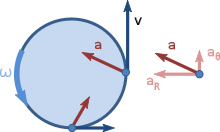Figure 5: Velocity and acceleration for nonuniform circular motion: the velocity vector is tangential to the orbit, but the acceleration vector is not radially inward because of its tangential component aθ that increases the rate of rotation: dω / dt = | aθ| / R.

As a generalization of the uniform circular motion case, suppose the angular rate of rotation is not constant. The acceleration now has a tangential component, as shown in Figure 5. This case is used to demonstrate a derivation strategy based upon a polar coordinate system.

Let r(t) be a vector that describes the position of a point mass as a function of time. Since we are assuming circular motion, let r(t) = R·ur, where R is a constant (the radius of the circle) and ur is the unit vector pointing from the origin to the point mass. The direction of ur is described by θ, the angle between the x-axis and the unit vector, measured counterclockwise from the x-axis. The other unit vector for polar coordinates, uθ is perpendicular to ur and points in the direction of increasing θ. These polar unit vectors can be expressed in terms of Cartesian unit vectors in the x and y directions, denoted i and j respectively:

ur = cosθ i + sinθ j

and

uθ = -sinθ i + cosθ j.

We differentiate to find velocity:$\mathbf{v} = R \frac {\mathrm{d} \mathbf{u}_\mathrm{r}}{\mathrm{d}t} = R \frac {\mathrm{d}}{\mathrm{d}t} \left( \mathrm{cos}\ \theta \ \mathbf{i} + \mathrm{sin}\ \theta \ \mathbf{j}\right)$$= R \frac {d \theta} {dt} \left( -\mathrm{sin}\ \theta \ \mathbf{i} + \mathrm{cos}\ \theta \ \mathbf{j}\right)\,$$= R \frac{\mathrm{d}\theta}{\mathrm{d}t} \mathbf{u}_\mathrm{\theta} \,$$= \omega R \mathbf{u}_\mathrm{\theta} \,$

where ω is the angular velocity dθ/dt.

This result for the velocity matches expectations that the velocity should be directed tangential to the circle, and that the magnitude of the velocity should be ωR. Differentiating again, and noting that${\frac {\mathrm{d}\mathbf{u}_\mathrm{\theta}}{\mathrm{d}t} = -\frac{\mathrm{d}\theta}{\mathrm{d}t} \mathbf{u}_\mathrm{r} = - \omega \mathbf{u}_\mathrm{r}} \ ,$

we find that the acceleration, a is:$\mathbf{a} = R \left( \frac {\mathrm{d}\omega}{\mathrm{d}t} \mathbf{u}_\mathrm{\theta} - \omega^2 \mathbf{u}_\mathrm{r} \right) \ .$

Thus, the radial and tangential components of the acceleration are:$\mathbf{a}_{\mathrm{r}} = - \omega^{2} R \ \mathbf{u}_\mathrm{r} = - \frac{|\mathbf{v}|^{2}}{ R} \ \mathbf{u}_\mathrm{r} \$   and$\ \mathbf{a}_{\mathrm{\theta}} = R \ \frac {\mathrm{d}\omega}{\mathrm{d}t} \ \mathbf{u}_\mathrm{\theta} = \frac {\mathrm{d} | \mathbf{v} | }{\mathrm{d}t} \ \mathbf{u}_\mathrm{\theta} \ ,$

where |v| = Rω is the magnitude of the velocity (the speed).

These equations express mathematically that, in the case of an object that moves along a circular path with a changing speed, the acceleration of the body may be decomposed into a perpendicular component that changes the direction of motion (the centripetal acceleration), and a parallel, or tangential component, that changes the speed.

### General planar motion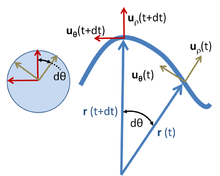Figure 6: Polar unit vectors at two times t and t + dt for a particle with trajectory r ( t ); on the left the unit vectors uρ and uθ at the two times are moved so their tails all meet, and are shown to trace an arc of a unit radius circle. Their rotation in time dt is dθ, just the same angle as the rotation of the trajectory r ( t ).

#### Polar coordinates

The above results can be derived perhaps more simply in polar coordinates, and at the same time extended to general motion within a plane, as shown next. Polar coordinates in the plane employ a radial unit vector uρ and an angular unit vector uθ, as shown in Figure 6. A particle at position r is described by:$\mathbf{r} = \rho \mathbf{u}_{\rho} \ ,$

where the notation ρ is used to describe the distance of the path from the origin instead of R to emphasize that this distance is not fixed, but varies with time. The unit vector uρ travels with the particle and always points in the same direction as r(t). Unit vector uθ also travels with the particle and stays orthogonal to uρ. Thus, uρ and uθ form a local Cartesian coordinate system attached to the particle, and tied to the path traveled by the particle. By moving the unit vectors so their tails coincide, as seen in the circle at the left of Figure 6, it is seen that uρ and uθ form a right-angled pair with tips on the unit circle that trace back and forth on the perimeter of this circle with the same angle θ(t) as r(t).

When the particle moves, its velocity is$\mathbf{v} = \frac {\mathrm{d} \rho }{\mathrm{d}t} \mathbf{u}_{\rho} + \rho \frac {\mathrm{d} \mathbf{u}_{\rho}}{\mathrm{d}t} \ .$

To evaluate the velocity, the derivative of the unit vector uρ is needed. Because uρ is a unit vector, its magnitude is fixed, and it can change only in direction, that is, its change duρ has a component only perpendicular to uρ. When the trajectory r(t) rotates an amount dθ, uρ, which points in the same direction as r(t), also rotates by dθ. See Figure 6. Therefore the change in uρ is$\mathrm{d} \mathbf{u}_{\rho} = \mathbf{u}_{\theta} \mathrm{d}\theta \ ,$

or$\frac {\mathrm{d} \mathbf{u}_{\rho}}{\mathrm{d}t} = \mathbf{u}_{\theta} \frac {\mathrm{d}\theta}{\mathrm{d}t} \ .$

In a similar fashion, the rate of change of uθ is found. As with uρ, uθ is a unit vector and can only rotate without changing size. To remain orthogonal to uρ while the trajectory r(t) rotates an amount dθ, uθ, which is orthogonal to r(t), also rotates by dθ. See Figure 6. Therefore, the change duθ is orthogonal to uθ and proportional to dθ (see Figure 6):$\frac{\mathrm{d} \mathbf{u}_{\theta}}{\mathrm{d}t} = -\frac {\mathrm{d} \theta} {\mathrm{d}t} \mathbf{u}_{\rho} \ .$

Figure 6 shows the sign to be negative: to maintain orthogonality, if duρ is positive with dθ, then duθ must decrease.

Substituting the derivative of uρ into the expression for velocity:$\mathbf{v} = \frac {\mathrm{d} \rho }{\mathrm{d}t} \mathbf{u}_{\rho} + \rho \mathbf{u}_{\theta} \frac {\mathrm{d} \theta} {\mathrm{d}t} = \mathbf{v}_{\rho} + \mathbf{v}_{\theta} \ .$

To obtain the acceleration, another time differentiation is done:$\mathbf{a} = \frac {\mathrm{d}^2 \rho }{\mathrm{d}t^2} \mathbf{u}_{\rho} + \frac {\mathrm{d} \rho }{\mathrm{d}t} \frac{\mathrm{d} \mathbf{u}_{\rho}}{\mathrm{d}t} + \frac {\mathrm{d} \rho}{\mathrm{d}t} \mathbf{u}_{\theta} \frac {\mathrm{d} \theta} {\mathrm{d}t} + \rho \frac{\mathrm{d} \mathbf{u}_{\theta}}{\mathrm{d}t} \frac {\mathrm{d} \theta} {\mathrm{d}t} + \rho \mathbf{u}_{\theta} \frac {\mathrm{d}^2 \theta} {\mathrm{d}t^2} \ .$

Substituting the derivatives of uρ and uθ, the acceleration of the particle is:$\mathbf{a} = \frac {\mathrm{d}^2 \rho }{\mathrm{d}t^2} \mathbf{u}_{\rho} + 2\frac {\mathrm{d} \rho}{\mathrm{d}t} \mathbf{u}_{\theta} \frac {\mathrm{d} \theta} {\mathrm{d}t}-\rho \mathbf{u}_{\rho}\left( \frac {\mathrm{d} \theta} {\mathrm{d}t}\right)^2 + \rho \mathbf{u}_{\theta} \frac {\mathrm{d}^2 \theta} {\mathrm{d}t^2} \ ,$$= \mathbf{u}_{\rho} \left[ \frac {\mathrm{d}^2 \rho }{\mathrm{d}t^2}-\rho\left( \frac {\mathrm{d} \theta} {\mathrm{d}t}\right)^2 \right] + \mathbf{u}_{\theta}\left[ 2\frac {\mathrm{d} \rho}{\mathrm{d}t} \frac {\mathrm{d} \theta} {\mathrm{d}t} + \rho \frac {\mathrm{d}^2 \theta} {\mathrm{d}t^2}\right] \$$= \mathbf{u}_{\rho} \left[ \frac {\mathrm{d}|\mathbf{v}_{\rho}|}{\mathrm{d}t}-\frac{|\mathbf{v}_{\theta}|^2}{\rho}\right] + \mathbf{u}_{\theta}\left[ \frac{2}{\rho}|\mathbf{v}_{\rho}||\mathbf{v}_{\theta}| + \rho\frac{\mathrm{d}}{\mathrm{d}t}\frac{|\mathbf{v}_{\theta}|}{\rho}\right] \ .$

As a particular example, if the particle moves in a circle of constant radius R, then dρ/dt = 0, v = vθ, and:$\mathbf{a} = \mathbf{u}_{\rho} \left[ -\rho\left( \frac {\mathrm{d} \theta} {\mathrm{d}t}\right)^2 \right] + \mathbf{u}_{\theta}\left[ \rho \frac {\mathrm{d}^2 \theta} {\mathrm{d}t^2}\right] \$$= \mathbf{u}_{\rho} \left[ -\frac{|\mathbf{v}|^2}{R}\right] + \mathbf{u}_{\theta}\left[ \frac {\mathrm{d} |\mathbf{v}|} {\mathrm{d}t}\right] \ .$

These results agree with those above for nonuniform circular motion. See also the article on non-uniform circular motion. If this acceleration is multiplied by the particle mass, the leading term is the centripetal force and the negative of the second term related to angular acceleration is sometimes called the Euler force.

For trajectories other than circular motion, for example, the more general trajectory envisioned in Figure 6, the instantaneous center of rotation and radius of curvature of the trajectory are related only indirectly to the coordinate system defined by uρ and uθ and to the length |r(t)| = ρ. Consequently, in the general case, it is not straightforward to disentangle the centripetal and Euler terms from the above general acceleration equation.  To deal directly with this issue, local coordinates are preferable, as discussed next.

#### Local coordinates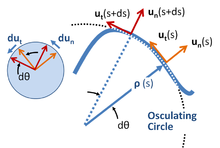Figure 7: Local coordinate system for planar motion on a curve. Two different positions are shown for distances s and s + ds along the curve. At each position s, unit vector un points along the outward normal to the curve and unit vector ut is tangential to the path. The radius of curvature of the path is ρ as found from the rate of rotation of the tangent to the curve with respect to arc length, and is the radius of the osculating circle at position s. The unit circle on the left shows the rotation of the unit vectors with s.

By local coordinates is meant a set of coordinates that travel with the particle,  and have orientation determined by the path of the particle. Unit vectors are formed as shown in Figure 7, both tangential and normal to the path. This coordinate system sometimes is referred to as intrinsic or path coordinates or nt-coordinates, for normal-tangential, referring to these unit vectors. These coordinates are a very special example of a more general concept of local coordinates from the theory of differential forms.

Distance along the path of the particle is the arc length s, considered to be a known function of time.$s = s(t) \ .$

A center of curvature is defined at each position s located a distance ρ (the radius of curvature) from the curve on a line along the normal un (s). The required distance ρ(s) at arc length s is defined in terms of the rate of rotation of the tangent to the curve, which in turn is determined by the path itself. If the orientation of the tangent relative to some starting position is θ(s), then ρ(s) is defined by the derivative dθ/ds:$\frac{1} {\rho (s)} = \kappa (s) = \frac {\mathrm{d}\theta}{\mathrm{d}s}\ .$

The radius of curvature usually is taken as positive (that is, as an absolute value), while the curvature κ is a signed quantity.

A geometric approach to finding the center of curvature and the radius of curvature uses a limiting process leading to the osculating circle. See Figure 7.

Using these coordinates, the motion along the path is viewed as a succession of circular paths of ever-changing center, and at each position s constitutes non-uniform circular motion at that position with radius ρ. The local value of the angular rate of rotation then is given by:$\omega(s) = \frac{\mathrm{d}\theta}{\mathrm{d}t} = \frac{\mathrm{d}\theta}{\mathrm{d}s} \frac {\mathrm{d}s}{\mathrm{d}t} = \frac{1}{\rho(s)}\ \frac {\mathrm{d}s}{\mathrm{d}t} = \frac{v(s)}{\rho(s)}\ ,$

with the local speed v given by:$v(s) = \frac {\mathrm{d}s}{\mathrm{d}t}\ .$

As for the other examples above, because unit vectors cannot change magnitude, their rate of change is always perpendicular to their direction (see the left-hand insert in Figure 7):$\frac{d\mathbf{u}_\mathrm{n}(s)}{ds} = \mathbf{u}_\mathrm{t}(s)\frac{d\theta}{ds} = \mathbf{u}_\mathrm{t}(s)\frac{1}{\rho} \ ;$$\frac{d\mathbf{u}_\mathrm{t}(s)}{\mathrm{d}s} = -\mathbf{u}_\mathrm{n}(s)\frac{\mathrm{d}\theta}{\mathrm{d}s} = - \mathbf{u}_\mathrm{n}(s)\frac{1}{\rho} \ .$

Consequently, the velocity and acceleration are:$\mathbf{v}(t) = v \mathbf{u}_\mathrm{t}(s)\ ;$

and using the chain-rule of differentiation:$\mathbf{a}(t) = \frac{\mathrm{d}v}{\mathrm{d}t} \mathbf{u}_\mathrm{t}(s) - \frac{v^2}{\rho}\mathbf{u}_\mathrm{n}(s) \ ;$ with the tangential acceleration$\frac{\mathrm{\mathrm{d}}v}{\mathrm{\mathrm{d}}t} = \frac{\mathrm{d}v}{\mathrm{d}s}\ \frac{\mathrm{d}s}{\mathrm{d}t} = \frac{\mathrm{d}v}{\mathrm{d}s}\ v \ .$

In this local coordinate system the acceleration resembles the expression for nonuniform circular motion with the local radius ρ(s), and the centripetal acceleration is identified as the second term.

Extension of this approach to three dimensional space curves leads to the Frenet-Serret formulas.

##### Alternative approach

Looking at Figure 7, one might wonder whether adequate account has been taken of the difference in curvature between ρ(s) and ρ(s + ds) in computing the arc length as ds = ρ(s)dθ. Reassurance on this point can be found using a more formal approach outlined below. This approach also makes connection with the article on curvature.

To introduce the unit vectors of the local coordinate system, one approach is to begin in Cartesian coordinates and describe the local coordinates in terms of these Cartesian coordinates. In terms of arc length s let the path be described as:$\mathbf{r}(s) = \left[ x(s),\ y(s) \right] \ .$

Then an incremental displacement along the path ds is described by:$\mathrm{d}\mathbf{r}(s) = \left[ \mathrm{d}x(s),\ \mathrm{d}y(s) \right] = \left[ x'(s),\ y'(s) \right] \mathrm{d}s \ ,$

where primes are introduced to denote derivatives with respect to s. The magnitude of this displacement is ds, showing that:$\left[ x'(s)^2 + y'(s)^2 \right] = 1 \ .$    (Eq. 1)

This displacement is necessarily tangent to the curve at s, showing that the unit vector tangent to the curve is:$\mathbf{u}_\mathrm{t}(s) = \left[ x'(s), \ y'(s) \right] \ ,$

while the outward unit vector normal to the curve is$\mathbf{u}_\mathrm{n}(s) = \left[ y'(s),\ -x'(s) \right] \ ,$

Orthogonality can be verified by showing that the vector dot product is zero. The unit magnitude of these vectors is a consequence of Eq. 1. Using the tangent vector, the angle θ of the tangent to the curve is given by:$\sin \theta = \frac{y'(s)}{\sqrt{x'(s)^2 + y'(s)^2}} = y'(s) \ ;$   and$\cos \theta = \frac{x'(s)}{\sqrt{x'(s)^2 + y'(s)^2}} = x'(s) \ .$

The radius of curvature is introduced completely formally (without need for geometric interpretation) as:$\frac{1}{\rho} = \frac{\mathrm{d}\theta}{\mathrm{d}s}\ .$

The derivative of θ can be found from that for sinθ:$\frac{\mathrm{d} \sin\theta}{\mathrm{d}s} = \cos \theta \frac {\mathrm{d}\theta}{\mathrm{d}s} = \frac{1}{\rho} \cos \theta \ = \frac{1}{\rho} x'(s)\ .$

Now:$\frac{\mathrm{d} \sin \theta }{\mathrm{d}s} = \frac{\mathrm{d}}{\mathrm{d}s} \frac{y'(s)}{\sqrt{x'(s)^2 + y'(s)^2}}$$= \frac{y''(s)x'(s)^2-y'(s)x'(s)x''(s)} {\left(x'(s)^2 + y'(s)^2\right)^{3/2}}\ ,$

in which the denominator is unity. With this formula for the derivative of the sine, the radius of curvature becomes:$\frac {\mathrm{d}\theta}{\mathrm{d}s} = \frac{1}{\rho} = y''(s)x'(s) - y'(s)x''(s)\$$= \frac{y''(s)}{x'(s)} = -\frac{x''(s)}{y'(s)} \ ,$

where the equivalence of the forms stems from differentiation of Eq. 1:$x'(s)x''(s) + y'(s)y''(s) = 0 \ .$

With these results, the acceleration can be found:$\mathbf{a}(s) = \frac{\mathrm{d}}{\mathrm{d}t}\mathbf{v}(s)$$= \frac{\mathrm{d}}{\mathrm{d}t}\left[\frac{\mathrm{d}s}{\mathrm{d}t} \left( x'(s), \ y'(s) \right) \right]\$$= \left(\frac{\mathrm{d}^2s}{\mathrm{d}t^2}\right)\mathbf{u}_\mathrm{t}(s) + \left(\frac{\mathrm{d}s}{\mathrm{d}t}\right) ^2 \left(x''(s),\ y''(s) \right)$$= \left(\frac{\mathrm{d}^2s}{\mathrm{d}t^2}\right)\mathbf{u}_\mathrm{t}(s) - \left(\frac{\mathrm{d}s}{\mathrm{d}t}\right) ^2 \frac{1}{\rho} \mathbf{u}_\mathrm{n}(s) \ ,$

as can be verified by taking the dot product with the unit vectors ut(s) and un(s). This result for acceleration is the same as that for circular motion based on the radius ρ. Using this coordinate system in the inertial frame, it is easy to identify the force normal to the trajectory as the centripetal force and that parallel to the trajectory as the tangential force. From a qualitative standpoint, the path can be approximated by an arc of a circle for a limited time, and for the limited time a particular radius of curvature applies, the centrifugal and Euler forces can be analyzed on the basis of circular motion with that radius.

This result for acceleration agrees with that found earlier. However, in this approach the question of the change in radius of curvature with s is handled completely formally, consistent with a geometric interpretation, but not relying upon it, thereby avoiding any questions Figure 7 might suggest about neglecting the variation in ρ.

##### Example: circular motion

To illustrate the above formulas, let x, y be given as:$x = \alpha \cos \frac{s}{\alpha} \ ; \ y = \alpha \sin\frac{s}{\alpha} \ .$

Then:$x^2 + y^2 = \alpha^2 \ ,$

which can be recognized as a circular path around the origin with radius α. The position s = 0 corresponds to [α, 0], or 3 o'clock. To use the above formalism the derivatives are needed:$y^{\prime}(s) = \cos \frac{s}{\alpha} \ ; \ x^{\prime}(s) = -\sin \frac{s}{\alpha} \ ,$$y^{\prime\prime}(s) = -\frac{1}{\alpha}\sin\frac{s}{\alpha} \ ; \ x^{\prime\prime}(s) = -\frac{1}{\alpha}\cos \frac{s}{\alpha} \ .$

With these results one can verify that:$x^{\prime}(s)^2 + y^{\prime}(s)^2 = 1 \ ; \ \frac{1}{\rho} = y^{\prime\prime}(s)x^{\prime}(s)-y^{\prime}(s)x^{\prime\prime}(s) = \frac{1}{\alpha} \ .$

The unit vectors also can be found:$\mathbf{u}_\mathrm{t}(s) = \left[-\sin\frac{s}{\alpha} \ , \ \cos\frac{s}{\alpha} \right] \ ; \ \mathbf{u}_\mathrm{n}(s) = \left[\cos\frac{s}{\alpha} \ , \ \sin\frac{s}{\alpha} \right] \ ,$

which serve to show that s = 0 is located at position [ρ, 0] and s = ρπ/2 at [0, ρ], which agrees with the original expressions for x and y. In other words, s is measured counterclockwise around the circle from 3 o'clock. Also, the derivatives of these vectors can be found:$\frac{\mathrm{d}}{\mathrm{d}s}\mathbf{u}_\mathrm{t}(s) = -\frac{1}{\alpha} \left[\cos\frac{s}{\alpha} \ , \ \sin\frac{s}{\alpha} \right] = -\frac{1}{\alpha}\mathbf{u}_\mathrm{n}(s) \ ;$$\ \frac{\mathrm{d}}{\mathrm{d}s}\mathbf{u}_\mathrm{n}(s) = \frac{1}{\alpha} \left[-\sin\frac{s}{\alpha} \ , \ \cos\frac{s}{\alpha} \right] = \frac{1}{\alpha}\mathbf{u}_\mathrm{t}(s) \ .$

To obtain velocity and acceleration, a time-dependence for s is necessary. For counterclockwise motion at variable speed v(t):$s(t) = \int_0^t \ dt^{\prime} \ v(t^{\prime}) \ ,$

where v(t) is the speed and t is time, and s(t = 0) = 0. Then:$\mathbf{v} = v(t)\mathbf{u}_\mathrm{t}(s) \ ,$$\mathbf{a} = \frac{\mathrm{d}v}{\mathrm{d}t}\mathbf{u}_\mathrm{t}(s) + v\frac{\mathrm{d}}{\mathrm{d}t}\mathbf{u}_\mathrm{t}(s) = \frac{\mathrm{d}v}{\mathrm{d}t}\mathbf{u}_\mathrm{t}(s)-v\frac{1}{\alpha}\mathbf{u}_\mathrm{n}(s)\frac{\mathrm{d}s}{\mathrm{d}t}$$\mathbf{a} = \frac{\mathrm{d}v}{\mathrm{d}t}\mathbf{u}_\mathrm{t}(s)-\frac{v^2}{\alpha}\mathbf{u}_\mathrm{n}(s) \ ,$

where it already is established that α = ρ. This acceleration is the standard result for non-uniform circular motion.

## Notes and references

1. ^  , Extract of page 291
2. ^ Russelkl C Hibbeler (2009). "Equations of Motion: Normal and tangential coordinates". Engineering Mechanics: Dynamics (12 ed.). Prentice Hall. p. 131. ISBN 0136077919.
3. ^ Paul Allen Tipler, Gene Mosca (2003). Physics for scientists and engineers (5th ed.). Macmillan. p. 129. ISBN 0716783398.
4. ^ Felix Klein, Arnold Sommerfeld (2008). The Theory of the Top (Reprint with translators' notes of 1897 ed.). Boston, Mass.: Birkhäuser. p. 232. ISBN 0817647201.
5. ^ Chris Carter (2001). Facts and Practice for A-Level: Physics. S.l.: Oxford Univ Press. p. 30. ISBN 9780199147687.
6. ^ Eugene Lommel and George William Myers (1900). Experimental physics. K. Paul, Trench, Trübner & Co. p. 63.
7. ^ Colwell, Catharine H.. "A Derivation of the Formulas for Centripetal Acceleration". PhysicsLAB. Retrieved 31 July 2011.
8. ^ Theo Koupelis (2010). In Quest of the Universe (6th ed.). Jones & Bartlett Learning. p. 83. ISBN 9780763768584.
9. ^ Johnnie T. Dennis (2003). The Complete Idiot's Guide to Physics. Alpha Books. p. 91. ISBN 9781592570812.
10. ^ A. V. Durrant (1996). Vectors in physics and engineering. CRC Press. p. 103. ISBN 9780412627101.
11. ^ Lawrence S. Lerner (1997). Physics for Scientists and Engineers. Boston: Jones & Bartlett Publishers. p. 128. ISBN 0867204796.
12. ^ Arthur Beiser (2004). Schaum's Outline of Applied Physics. New York: McGraw-Hill Professional. p. 103. ISBN 0071426116.
13. ^ Alan Darbyshire (2003). Mechanical Engineering: BTEC National Option Units. Oxford: Newnes. p. 56. ISBN 0750657618.
14. ^ Federal Aviation Administration (2007). Pilot's Encyclopedia of Aeronautical Knowledge. Oklahoma City OK: Skyhorse Publishing Inc.. Figure 3–21. ISBN 1602390347.
15. ^ Note: unlike the Cartesian unit vectors i and j, which are constant, in polar coordinates the direction of the unit vectors ur and uθ depend on θ, and so in general have non-zero time derivatives.
16. ^ Although the polar coordinate system moves with the particle, the observer does not. The description of the particle motion remains a description from the stationary observer's point of view.
17. ^ Notice that this local coordinate system is not autonomous; for example, its rotation in time is dictated by the trajectory traced by the particle. Note also that the radial vector r(t) does not represent the radius of curvature of the path.
18. ^ John Robert Taylor (2005). Classical Mechanics. Sausalito CA: University Science Books. pp. 28–29. ISBN 189138922X.
19. ^ Cornelius Lanczos (1986). The Variational Principles of Mechanics. New York: Courier Dover Publications. p. 103. ISBN 0486650677.
20. ^ See, for example, Howard D. Curtis (2005). Orbital Mechanics for Engineering Students. Butterworth-Heinemann. p. 5. ISBN 0750661690.
21. ^ S. Y. Lee (2004). Accelerator physics (2nd ed.). Hackensack NJ: World Scientific. p. 37. ISBN 981256182X.
22. ^ The observer of the motion along the curve is using these local coordinates to describe the motion from the observer's frame of reference, that is, from a stationary point of view. In other words, although the local coordinate system moves with the particle, the observer does not. A change in coordinate system used by the observer is only a change in their description of observations, and does not mean that the observer has changed their state of motion, and vice versa.
23. ^ Zhilin Li & Kazufumi Ito (2006). The immersed interface method: numerical solutions of PDEs involving interfaces and irregular domains. Philadelphia: Society for Industrial and Applied Mathematics. p. 16. ISBN 0898716098.
24. ^ K L Kumar (2003). Engineering Mechanics. New Delhi: Tata McGraw-Hill. p. 339. ISBN 0070494738.
25. ^ Lakshmana C. Rao, J. Lakshminarasimhan, Raju Sethuraman & SM Sivakuma (2004). Engineering Dynamics: Statics and Dynamics. Prentice Hall of India. p. 133. ISBN 8120321898.
26. ^ Shigeyuki Morita (2001). Geometry of Differential Forms. American Mathematical Society. p. 1. ISBN 0821810456.
27. ^ The osculating circle at a given point P on a curve is the limiting circle of a sequence of circles that pass through P and two other points on the curve, Q and R, on either side of P, as Q and R approach P. See the online text by Lamb: Horace Lamb (1897). An Elementary Course of Infinitesimal Calculus. University Press. p. 406. ISBN 1108005349.
28. ^ a b Guang Chen & Fook Fah Yap (2003). An Introduction to Planar Dynamics (3rd ed.). Central Learning Asia/Thomson Learning Asia. p. 34. ISBN 9812435689.
29. ^ R. Douglas Gregory (2006). Classical Mechanics: An Undergraduate Text. Cambridge University Press. p. 20. ISBN 0521826780.
30. ^ Edmund Taylor Whittaker & William McCrea (1988). A Treatise on the Analytical Dynamics of Particles and Rigid Bodies: with an introduction to the problem of three bodies (4rth ed.). Cambridge University Press. p. 20. ISBN 0521358833.
31. ^ Jerry H. Ginsberg (2007). Engineering Dynamics. Cambridge University Press. p. 33. ISBN 0521883032.
32. ^ Joseph F. Shelley (1990). 800 solved problems in vector mechanics for engineers: Dynamics. McGraw-Hill Professional. p. 47. ISBN 0070566879.
33. ^ Larry C. Andrews & Ronald L. Phillips (2003). Mathematical Techniques for Engineers and Scientists. SPIE Press. p. 164. ISBN 0819445061.
34. ^ Ch V Ramana Murthy & NC Srinivas (2001). Applied Mathematics. New Delhi: S. Chand & Co.. p. 337. ISBN 81-219-2082-5.
35. ^ The article on curvature treats a more general case where the curve is parametrized by an arbitrary variable (denoted t), rather than by the arc length s.
36. ^ Ahmed A. Shabana, Khaled E. Zaazaa, Hiroyuki Sugiyama (2007). Railroad Vehicle Dynamics: A Computational Approach. CRC Press. p. 91. ISBN 1420045814.

• Serway, Raymond A.; Jewett, John W. (2004). Physics for Scientists and Engineers (6th ed.). Brooks/Cole. ISBN 0-534-40842-7.
• Tipler, Paul (2004). Physics for Scientists and Engineers: Mechanics, Oscillations and Waves, Thermodynamics (5th ed.). W. H. Freeman. ISBN 0-7167-0809-4.
• Centripetal force vs. Centrifugal force, from an online Regents Exam physics tutorial by the Oswego City School District

Wikimedia Foundation. 2010.

### Look at other dictionaries:

• Centripetal force — Force Force, n. [F. force, LL. forcia, fortia, fr. L. fortis strong. See {Fort}, n.] 1. Capacity of exercising an influence or producing an effect; strength or energy of body or mind; active power; vigor; might; often, an unusual degree of… …   The Collaborative International Dictionary of English

• Centripetal force — Centripetal Cen*trip e*tal, a. [L. centrum center + petere to move toward.] 1. Tending, or causing, to approach the center. [1913 Webster] 2. (Bot.) (a) Expanding first at the base of the inflorescence, and proceeding in order towards the summit …   The Collaborative International Dictionary of English

• centripetal force — ► NOUN Physics ▪ a force which acts on a body moving in a circular path and is directed towards the centre around which the body is moving …   English terms dictionary

• centripetal force — n. the force tending to pull a thing toward the center of rotation when it is rotating around a center …   English World dictionary

• centripetal force — noun Date: 1686 the force that is necessary to keep an object moving in a curved path and that is directed inward toward the center of rotation < a string on the end of which a stone is whirled about exerts centripetal force on the stone >… …   New Collegiate Dictionary

• centripetal force — UK [senˌtrɪpɪt(ə)l ˈfɔː(r)s] / US [senˌtrɪpət(ə)l ˈfɔrs] noun [uncountable] physics a force that makes things move towards the centre of something when they are moving around that centre. Gravity is the centripetal force that keeps the planets… …   English dictionary

• centripetal force — įcentrinė jėga statusas T sritis Standartizacija ir metrologija apibrėžtis Kreivai judančią dalelę arba materialųjį tašką veikianti jėga, nukreipta pagal jos trajektorijos pagrindinę normalę į kreivumo centrą. atitikmenys: angl. centripetal force …   Penkiakalbis aiškinamasis metrologijos terminų žodynas

• centripetal force — įcentrinė jėga statusas T sritis fizika atitikmenys: angl. centripetal force vok. Zentripetalkraft, f rus. центростремительная сила, f pranc. force centripète, f …   Fizikos terminų žodynas

• centripetal force — force which pulls objects toward the center around which they are moving …   English contemporary dictionary

• centripetal force — /sɛnˌtrɪpətl ˈfɔs/ (say sen.tripuhtl faws) noun Physics a force, directed towards the centre of a circle or curve, which causes a body to move in a circular or curved path. Also, centripetal action …   Australian English dictionary